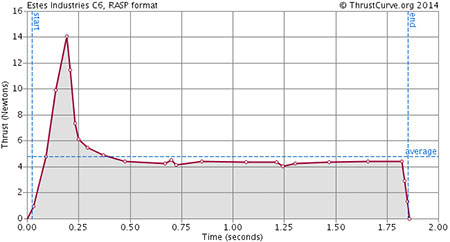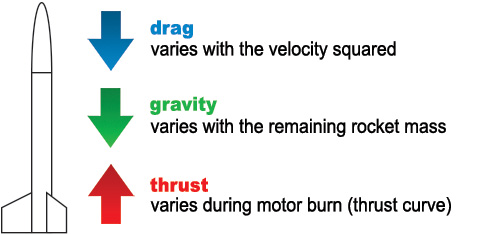HOWTO

# Flight Physics

Most people are satisfied to use a flight simulator to design their rockets and get a prediction of the stability and performance from a design. This page goes through some of the background that goes into simulation, which is helpful to make sense of the concepts used in the sport.

The major forces acting on a rocket are: motor thrust, drag and gravity. Drag is poorly understood and for someone who wants to optimize a rocket for speed (Mach-busting) or altitude, understanding how it affects performance is essential.

This page will use some math, for those interested in the details, but I hope it's also informative to those who aren't interested in looking at equations. In general, the details of the equations aren't critical, you can skip over them to get the gist from the text and demonstration, but you should at least try to understand them (they're not too complex).

I've used S.I. units everywhere for consistency, but all the same math works with imperial units (just with messier conversions). As you go through the calculations, you'll see how easy they are with S.I. units and that it's best to do them this way, then convert to imperial at the end if desired.

## The Forces

So, what's actually going on when we fly a rocket? Gravity and motor thrust are the two most obvious forces, so let's look at how they affect the rocket. Gravity applies a constant acceleration of (approximately) 10m/s². Your rocket motor applies a force as it burns, countering gravity.

You've no doubt seen this famous equation (Newton's second law of motion), spoken as "force (F) equals mass (m) times acceleration (a)":

F = m a

• F force, measured in newtons (N)
• m mass, the amount of stuff (weight), measured in kilograms (Kg)
• a acceleration, how fast the velocity (speed) is changing (m/s²)

This law is how we relate acceleration and mass to force. The newton (unit of force) is 1 Kg m/s² (kilogram × acceleration). For those who prefer imperial units, 1N = 0.225lbf.

### Thrust

The force produced by the rocket motor varies during its burn, and this profile of force over time is called "the thrust curve". You need this data to run a simulation (see ThrustCurve.org for more info). For example, this is the thrust curve of the Estes C6. Note how the thrust varies over the 1.9 second burn time, with a high of 14N, but an average of 5N.FT = f(t)

• FT force from motor thrust
• f(t) function of time (thrust curve)
• t time since motor ignition (seconds)

### Gravity

Accelerations, like gravity, are measured in m/s² (meters per second, per second). We take gravitational attraction to be a constant for our purposes (near the Earth's surface):

FG = m g

• FG force applied by gravity
• m the remaining mass of the rocket
• g 9.8 m/s²

Gravity acts as an acceleration; to calculate the force we need to know the mass. The mass is that of the rocket plus the motor, but as the motor burns its mass decreases. (This is why both the total and propellant weights masses are included with simulator data.)

### Drag

The non-obvious, but critical, force is drag. Rockets fly through the air at a high speed, and to do so, they must push the air molecules out of the way. Drag is a more complex equation:

FD = ½ ρ v² CD A

• FD is the force produced by drag
• ρ (Greek letter 'rho') is the air density, which decreases with altitude
• drag is proportional to the square of the velocity (speed)
• CD coefficient of drag, accounting for the shape and smoothness of the rocket
• A is the frontal area of the rocket, usually circular (πr²)

Note that all this is even more complex for high-quality simulation, but for hobby purposes, the bigger unknowns swallow the finer technical details. Finally, this description is only valid for sub-sonic flights. Once speeds approach and exceed that of sound (Mach 1), the analysis becomes much different.

CD needs a little more explanation. This term has no dimensions, it is simply a number used to describe how the shape of the body and its angle to the wind influence drag. All shapes that move through the air posses drag coefficients. Finding the CD value for your rocket is critical for accurate simulations.* There are theoretical models and experimental methods for calculating it, but for hobby rocket simulation a simple approximation is generally used.

### All Together Now

So now we know how to calculate the forces that will affect the rocket in flight. Gravity is a force pulling the rocket down on the rocket's mass, thrust is the force produced by the burning rocket motor pushing it up, and drag is the force resisting its passage through air.## Flight Simulation

Flight simulators work by applying these forces to the rocket and calculating the net acceleration, current velocity and altitude. See ThrustCurve.org for a high-level explanation.

To simulate, we calculate instantaneous acceleration (a) at each point in time. We maintain a cumulative velocity (v) by integrating the acceleration, and a cumulative altitude (h) by integrating the velocity (starting from zero).

### Equations

First, instantaneous acceleration as a:

F = FT − FD − FG

a = F / m

• Fnet force
• ainstantaneous acceleration
• mremaining mass

Next, current velocity (speed) as v':

v' = v + a Δt

• v'updated velocity
• vprior velocity
• ainstantaneous acceleration
• Δttime since last update

And finally current altitude as h':

h' = h + v' Δt

• h'updated altitude
• hprior altitude
• v'updated velocity
• Δttime since last update

Iterating this process until h starts decreasing produces the statistics familar to users of simulators: maximum acceleration, maximum velocity and maximum altitude (apogee).

### Method

"Integration" is simply breaking the time down into tiny steps and treating varying forces as a constant during each time period. For example, the toy simulator below breaks the time down into 1/100ths of a second. This time step can be made arbitrarily small to achieve any desired accuracy.

Initially, we set velocity (v) and altitude (h) to zero and liftoff detected to false. Also, we set the mass (m) to that of the rocket plus motor. Then we iterate, adjusting our cumulative values by the instantaneous forces for that time period. Let's assume our rocket has an initial mass of 10g (0.01Kg).

FT = f(t) = 0

FD = ½ ρ v² CD A = 0

FG = m g = 0.098

F = FT − FD − FG = −0.098

a = F / m = −9.8

At this point, we have not yet detected liftoff, so we don't update v or h. (If we did, the rocket would move down because the only non-zero acceleration is from gravity.) Once we do detect liftoff, we can start calculating v and h by integrating a and v. Let's assume we are working in 1/10th of a second steps (Δt = 0.1), and the motor has an average thrust of 8N during the first 1/10th second:

t = 0.1

FT = f(t) = 8

FD = ½ ρ v² CD A = 0

FG = m g = 0.098

F = FT − FD − FG = 7.9

a = F / m = 79

Now we see a positive acceleration, which means liftoff is detected, and we can begin accumulating velocity and altitude. I've included the units here because they help show how integration works and how closely the three measures are related:

a = 79 m/s²

Δt = 0.1s

v' = v + a Δt = 7.9 m/s

h' = h + v' Δt = 0.79 m

This process continues until apogee is detected. Note that this toy simulator stops at apogee, but full-featured flight simulators also simulate the behavior during descent (as well as having a more complex drag model and handling a variety of launch conditions).

### Illustration

I've implemented a simple simulator right on this page (in JavaScript). For the curious, you can view the source of this page to get the code. There are two familar rockets and two motors to try:

Rocket
Choose:
Diameter:
Mass:
CD:
Motor
Choose:
Impulse:
Mass:
Burn Time:

Launch Info
Liftoff Mass:
Time to Liftoff:
Rod Length:
Time on Rod:
Velocity off Rod:
Flight Info
Max Accel:
Max Velocity:
Max Altitude:
Time to Apogee:
Optimal Delay:

Note in the chart above how the force of drag is nearly as strong as the motor thrust towards the end of the burn, when maximum velocity has been reached (particularly for the Mosquito).

This chart has three separate plots; click on the labels to see acceleration, velocity and altitude during the flight. Flip back and forth between the plots to see how the integration works (acceleration → velocity → altitude).

Drag (air resistance) is worth further reading.

Air density (ρ) is more complex than one would think, varying not only with altitude but temperature and other factors.

The Karman line is the conventional end of the atmosphere/beginning of space.

The definition of acceleration is important for understanding interaction of force, mass and velocity.

Newton's laws of motion are fundamental to rocket flight.

Integration is a key operation in calculus.

A couple of relevant Estes technical reports: TR-10: Altitude Prediction Charts and TR-11: Aerodynamic Drag of Model Rockets.
* Parts of the description of CD are taken from these papers.

Randy Culp put together similar pages, one on flight simulation and one on rocketry equations.